Updating search results...

# 10 Results

View
Selected filters:
• OH.TMM.0005.Core.1Rating
0.0 stars

The Calculus I course was developed through the Ohio Department of Higher Education OER Innovation Grant. This work was completed and the course was posted in February 2019.&nbsp;The course is part of the Ohio Transfer Module and is also named TMM005. For more information about credit transfer between Ohio colleges and universities, please visit: www.ohiohighered.org/transfer.Team LeadJim Fowler &nbsp; &nbsp; &nbsp; &nbsp; &nbsp; &nbsp; &nbsp; &nbsp; &nbsp; &nbsp;&nbsp;&nbsp;&nbsp;&nbsp;&nbsp;&nbsp;&nbsp;&nbsp;&nbsp;&nbsp;&nbsp;&nbsp;&nbsp;&nbsp;&nbsp;&nbsp;&nbsp;&nbsp;&nbsp;&nbsp; Ohio State UniversityRita Ralph&nbsp;&nbsp;&nbsp;&nbsp;&nbsp;&nbsp;&nbsp;&nbsp;&nbsp;&nbsp;&nbsp;&nbsp;&nbsp;&nbsp;&nbsp;&nbsp;&nbsp;&nbsp;&nbsp;&nbsp;&nbsp;&nbsp;&nbsp;&nbsp;&nbsp;&nbsp;&nbsp;&nbsp;&nbsp;&nbsp;&nbsp;&nbsp;&nbsp;&nbsp;&nbsp;&nbsp;&nbsp;&nbsp;&nbsp;&nbsp; Columbus State Community CollegeContent ContributorsNela Lakos &nbsp; &nbsp;&nbsp; &nbsp;&nbsp;&nbsp;&nbsp;&nbsp;&nbsp;&nbsp;&nbsp;&nbsp;&nbsp;&nbsp;&nbsp;&nbsp;&nbsp;&nbsp;&nbsp;&nbsp;&nbsp;&nbsp;&nbsp;&nbsp;&nbsp;&nbsp;&nbsp;&nbsp;&nbsp;&nbsp;&nbsp;&nbsp;&nbsp;&nbsp;&nbsp; Ohio State UniversityBart Snapp &nbsp; &nbsp; &nbsp; &nbsp; &nbsp; &nbsp; &nbsp;&nbsp; &nbsp;&nbsp;&nbsp;&nbsp;&nbsp;&nbsp;&nbsp;&nbsp;&nbsp;&nbsp;&nbsp;&nbsp;&nbsp;&nbsp;&nbsp;&nbsp;&nbsp;&nbsp;&nbsp;&nbsp;&nbsp;&nbsp; Ohio State UniversityJames Talamo&nbsp; &nbsp; &nbsp; &nbsp; &nbsp; &nbsp; &nbsp; &nbsp; &nbsp; &nbsp; &nbsp; &nbsp; &nbsp; &nbsp; &nbsp; &nbsp; &nbsp; Ohio State UniversityXiang Yan&nbsp;&nbsp;&nbsp;&nbsp;&nbsp;&nbsp;&nbsp;&nbsp;&nbsp;&nbsp;&nbsp;&nbsp;&nbsp;&nbsp;&nbsp;&nbsp;&nbsp;&nbsp;&nbsp;&nbsp;&nbsp;&nbsp;&nbsp;&nbsp;&nbsp;&nbsp;&nbsp;&nbsp;&nbsp;&nbsp;&nbsp;&nbsp;&nbsp;&nbsp;&nbsp;&nbsp;&nbsp;&nbsp;&nbsp;&nbsp; Edison State Community CollegeLibrarianDaniel Dotson&nbsp;&nbsp;&nbsp; &nbsp; &nbsp; &nbsp; &nbsp; &nbsp; &nbsp; &nbsp; &nbsp; &nbsp; &nbsp; &nbsp; &nbsp; &nbsp; &nbsp; &nbsp; &nbsp; Ohio State University&nbsp;&nbsp;&nbsp;&nbsp;&nbsp;&nbsp;&nbsp;&nbsp;&nbsp;&nbsp;&nbsp;&nbsp;&nbsp;&nbsp;&nbsp;&nbsp;&nbsp;&nbsp;&nbsp;&nbsp;&nbsp;Review TeamThomas Needham&nbsp;&nbsp;&nbsp;&nbsp;&nbsp;&nbsp;&nbsp;&nbsp;&nbsp;&nbsp;&nbsp;&nbsp;&nbsp;&nbsp;&nbsp;&nbsp;&nbsp;&nbsp;&nbsp;&nbsp;&nbsp;&nbsp;&nbsp;&nbsp;&nbsp;&nbsp;&nbsp;&nbsp; Ohio State UniversityCarl Stitz&nbsp;&nbsp;&nbsp;&nbsp;&nbsp;&nbsp;&nbsp;&nbsp;&nbsp;&nbsp;&nbsp;&nbsp;&nbsp;&nbsp;&nbsp;&nbsp;&nbsp;&nbsp;&nbsp;&nbsp;&nbsp;&nbsp;&nbsp;&nbsp;&nbsp;&nbsp;&nbsp;&nbsp;&nbsp;&nbsp;&nbsp;&nbsp;&nbsp;&nbsp;&nbsp;&nbsp;&nbsp;&nbsp;&nbsp;&nbsp;&nbsp;&nbsp;&nbsp; Lakeland Community CollegeSara Rollo &nbsp; &nbsp; &nbsp; &nbsp; &nbsp; &nbsp; &nbsp; &nbsp; &nbsp; &nbsp; &nbsp; &nbsp; &nbsp; &nbsp; &nbsp; &nbsp; &nbsp; &nbsp; &nbsp; &nbsp; &nbsp;North Central State College&nbsp;

Subject:
Calculus
Mathematics
Material Type:
Full Course
Provider:
Ohio Open Ed Collaborative
09/26/2018Conditional Remix & Share Permitted
CC BY-NC
Rating
0.0 stars

After completing this section, students should be able to do the following.Compute average velocity.Approximate instantaneous velocity.Compare average and instantaneous velocity.Compute instantaneous velocity.

Subject:
Calculus
Material Type:
Module
06/28/2019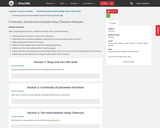Conditional Remix & Share Permitted
CC BY-NC
Rating
0.0 stars

After completing this section, students should be able to do the following.Identify where a function is, and is not, continuous.Understand the connection between continuity of a function and the value of a limit.Make a piecewise function continuous.State the Intermediate Value Theorem including hypotheses.Determine if the Intermediate Value Theorem applies.Sketch pictures indicating why the Intermediate Value Theorem is true, and why all hypotheses are necessary.Explain why certain points exist using the Intermediate Value Theorem.

Subject:
Calculus
Material Type:
Module
06/28/2019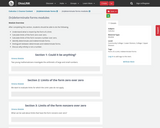Conditional Remix & Share Permitted
CC BY-NC
Rating
0.0 stars

After completing this section, students should be able to do the following.Understand what is meant by the form of a limit.Calculate limits of the form zero over zero.Calculate limits of the form nonzero number over zero.Identify determinate and indeterminate forms.Distinguish between determinate and indeterminate forms.Discuss why infinity is not a number.

Subject:
Calculus
Material Type:
Module
06/28/2019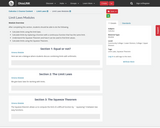Conditional Remix & Share Permitted
CC BY-NC
Rating
0.0 stars

After completing this section, students should be able to do the following.Calculate limits using the limit laws.Calculate limits by replacing a function with a continuous function that has the same limit.Understand the Squeeze Theorem and how it can be used to find limit values.Calculate limits using the Squeeze Theorem.

Subject:
Calculus
Material Type:
Module
06/28/2019Conditional Remix & Share Permitted
CC BY-NC
Rating
0.0 stars

After completing this section, students should be able to do the following.Understand the statement of the Extreme Value Theorem.Understand the statement of the Mean Value Theorem.Sketch pictures to illustrate why the Mean Value Theorem is true.Determine whether Rolle&rsquo;s Theorem or the Mean Value Theorem can be applied.Find the values guaranteed by Rolle&rsquo;s Theorem or the Mean Value Theorem.Use the Mean Value Theorem to solve word problems.Compare and contrast the Intermediate Value Theorem, Mean Value Theorem, and Rolle&rsquo;s Theorem.Identify calculus ideas which are consequences of the Mean Value Theorem.

Subject:
Calculus
Material Type:
Module
07/02/2019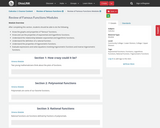Conditional Remix & Share Permitted
CC BY-NC
Rating
0.0 stars

After completing this section, students should be able to do the following.Know the graphs and properties of &lsquo;&lsquo;famous&rsquo;&rsquo; functions.Know and use the properties of exponential and logarithmic functions.Understand the relationship between exponential and logarithmic functions.Understand the definition of a rational function.Understand the properties of trigonometric functions.Evaluate expressions and solve equations involving trigonometric functions and inverse trigonometric functions.

Subject:
Calculus
Material Type:
Module
06/28/2019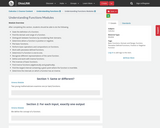Conditional Remix & Share Permitted
CC BY-NC
Rating
0.0 stars

After completing this section, students should be able to do the following.State the definition of a function.Find the domain and range of a function.Distinguish between functions by considering their domains.Determine where a function is positive or negative.Plot basic functions.Perform basic operations and compositions on functions.Work with piecewise defined functions.Determine if a function is one-to-one.Recognize different representations of the same function.Define and work with inverse functions.Plot inverses of basic functions.Find inverse functions (algebraically and graphically).Find the largest interval containing a given point where the function is invertible.Determine the intervals on which a function has an inverse.

Subject:
Calculus
Material Type:
Module
06/28/2019Conditional Remix & Share Permitted
CC BY-NC
Rating
0.0 stars

After completing this section, students should be able to do the following.Consider values of a function at inputs approaching a given point.Understand the concept of a limit.Use limits to understand local behavior of functions.Calculate limits from a graph (or state that the limit does not exist).Understand possible issues when estimating limits using nearby values.Define a one-sided limit.Explain the relationship between one-sided and two-sided limits.Distinguish between limit values and function values.Identify when a limit does not exist.Define continuity in terms of limits.Use the continuity of famous functions (on their domains) when computing limits.

Subject:
Calculus
Material Type:
Module
06/28/2019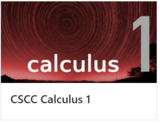Unrestricted Use
CC BY
Rating
0.0 stars

This is a Calculus I interactive textbook with modules curated and created on The Ohio State University's Ximera platform.

Subject:
Calculus
Mathematics
Material Type:
Textbook
Provider:
Columbus State Community College
Author:
Kevin James
Lee Wayand
Rita Ralph
The Ximera Project
Tzy-Yi "Alan" Yang